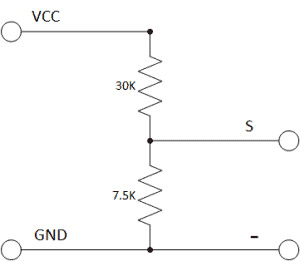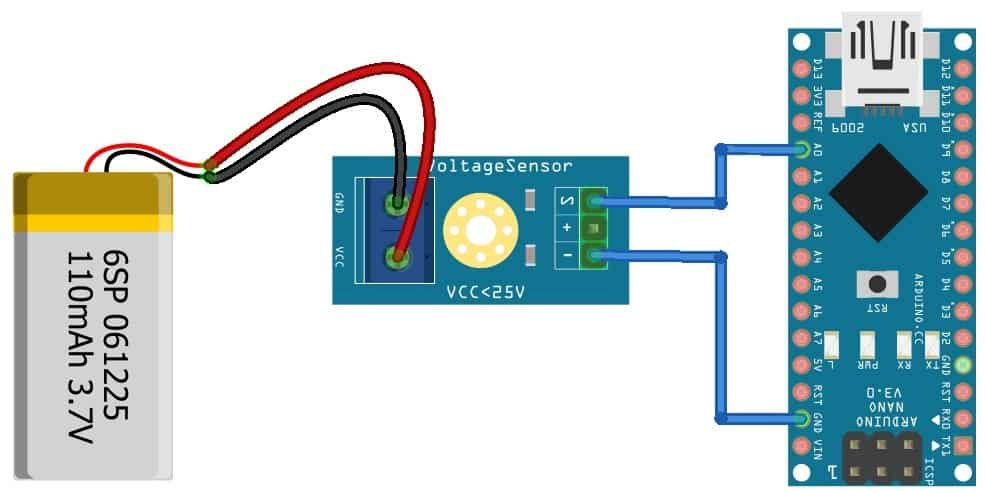## Voltage Sensor Module DC 0-25V

•••••Description:

The Voltage Sensor is basically a Voltage Divider consisting of two resistors with resistances of 30KΩ and 7.5KΩ i.e. a 5 to 1 voltage divider. Hence the output voltage is reduced by a factor of 5 for any input voltage . The internal circuit diagram of the Voltage Sensor Module is given below:Interfacing 0-25V DC Voltage Sensor Module with Arduino

Let us learn interfacing of Voltage Sensor Module with Arduino. The circuit diagram is given below.The connection is very simple. Connect the Signal (S) and Negative (-) pin of Voltage Sensor to Arduino A0 & GND pin respectively.

Note:

This module is based on principle of resistive voltage divider design, can make the red terminal connector input voltage to 5 times smaller.

DC 0-25V voltage sensor module input voltages up to 5v,the voltage detection module input voltage not greater than 5Vx5=25V (if using 3.3V systems, input voltage not greater than 3.3Vx5=16.5V)

Voltage tester sensor the minimum voltage of input voltage detection module is 0.00489Vx5=0.02445V.

This voltage detection sensor module DC 0-25V can work with analog single phase

Resolution: 0.00489V; input: Terminal cathode connected to VCC, GND negative pole; Output interface: "+" then 5/3.3V, "-" then GND, "s" then the for AD pins.

Features:

Voltage input range: DC 0-25 V

Voltage detection range: DC 0.02445 V-25 V

Voltage analog resolution: 0.00489V

Output Interface: "+ " connected 5/3.3V, "-" connected GND, "s" connected AD pins

DC input interface: red terminal positive with VCC, negative with GND

By 3P connector, connect this module with the expansion of board, not only makes it easier for you to detect voltage battery, can also use the IICLCD1602 LCD to display voltage

Kit include:

1 x Voltage Sensor Module

Mikroelectron Code:

`// Define analog input#define ANALOG_IN_PIN A0// Floats for ADC voltage & Input voltagefloat adc_voltage = 0.0;float in_voltage = 0.0; // Floats for resistor values in divider (in ohms)float R1 = 30000.0;float R2 = 7500.0;  // Float for Reference Voltagefloat ref_voltage = 5.0; // Integer for ADC valueint adc_value = 0; void setup(){   // Setup Serial Monitor   Serial.begin(9600);   Serial.println("DC Voltage Test");} void loop(){   // Read the Analog Input   adc_value = analogRead(ANALOG_IN_PIN);      // Determine voltage at ADC input   adc_voltage  = (adc_value * ref_voltage) / 1024.0;       // Calculate voltage at divider input   in_voltage = adc_voltage / (R2/(R1+R2)) ;       // Print results to Serial Monitor to 2 decimal places  Serial.print("Input Voltage = ");  Serial.println(in_voltage, 2);    // Short delay  delay(500);}`

1 JD
Quantity
In stock

## subscribe to our weekly newsletter